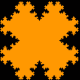# How to convert Array{Array{Float64, 1}, 1} to Matrix in julia?

### Tags:

#### julia

Let's say I have input of this kind:

``````> [[0.8681299566762923,-0.3472589826095631], [3.2300860990307445,3.3731249077464946]]
``````

How can I convert it to more pleasant type like Matrix (knowing dimensions)?983asked Mar 17 '23 23:03

#### Piotr Gródek

You can use splatting (`...`) and `hcat` to get what you are after:

``````julia> a = Vector[[0.8681299566762923,-0.3472589826095631], [3.2300860990307445,3.3731249077464946]]
2-element Array{Array{T,1},1}:
[0.8681299566762923,-0.3472589826095631]
[3.2300860990307445,3.3731249077464946]

julia> hcat(a...)
2x2 Array{Float64,2}:
0.86813   3.23009
-0.347259  3.37312
``````

Or if you wanted stop stack as rows instead of columns you could do the following:

``````julia> vcat(map(x->x', a)...)
2x2 Array{Float64,2}:
0.86813  -0.347259
3.23009   3.37312
``````

I don't recommend building a `Matrix` row by row as that is in conflict with Julia's column major array layout. For larger matrices it is actually more efficient to stack as columns and transpose the output:

``````julia> a2 = Vector{Float64}[rand(10) for i=1:5000];

julia> stackrows1{T}(a::Vector{Vector{T}}) = vcat(map(transpose, a)...)::Matrix{T}
stackrows1 (generic function with 2 methods)

julia> stackrows2{T}(a::Vector{Vector{T}}) = hcat(a...)'::Matrix{T}
stackrows2 (generic function with 2 methods)

julia> stackrows1(a2) == stackrows2(a2)  # run once to compile and make sure functions do the same thing
true

julia> @time for i=1:100 stackrows1(a2); end
elapsed time: 0.142792896 seconds (149 MB allocated, 7.85% gc time in 7 pauses with 0 full sweep)

julia> @time for i=1:100 stackrows2(a2); end
elapsed time: 0.05213114 seconds (88 MB allocated, 12.60% gc time in 4 pauses with 0 full sweep)
``````97answered Mar 24 '23 15:03